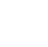Back to Chapter
Java IntroductionJava BasicsBranching and LoopingClasses, Objects, and MethodsArrays, Strings, and VectorsPackagesErrors and ExceptionApplet ProgrammingGraphics ProgrammingManaging I/O FilesJava Database Connectivity# Java Applet Programming Excercise

## Problem Statement-1:

Write a program in Java such that it accepts a number and displays the square of the number 12 using applet.  Create an input text field to accept a number from user. Create a button to confirm the number and calculate its square. When the button is clicked, display its square in a text field.

Test Data:

Enter a number : 12

Expected Output :

Enter a number : 12

Square of 12.0 is 144.0

Solution:

// Square_Num.java
import java.awt.*;
import java.applet.*;
import java.awt.event.*;
public class Square_Num extends Applet implements ActionListener
{
TextField num,out;
//Initialize with required input fields
public void init()
{
Label label = new Label("Enter a number :");
num = new TextField(5);
Button calc = new Button("Calculate");
out = new TextField();
}
//Function to display the square of the number
public void actionPerformed(ActionEvent e)
{
double n = Double.valueOf(num.getText());
double square=n*n;
out.setText("Square of "+ n +" is "+square);
revalidate();
}
}

//Square_Num.html
<html>
<title>Square root</title>
<applet code = Square_Num.class width=500 height=500>
</applet>
</html>

Output:

Explanation:

In the class Square_Num which is extended from the Applet Class declare  num, out in TextField type. TextField creates a text field in applet. The Button creates a button in applet. The calc is the object of button and it performs the addActionListener method.The double square=n*n is performed to get square root of the given number.